Next: Model Selecton Up: Statistical Background Previous: Least Squares Approaches

## Lagrange Techniques

A description of optimization measures would not be complete without some mention of the method first suggested by Lagrange. In some circumstances we require not only an optimal (maximum or minimum) of a statistical measure but also that a set of contraints on the parameter set be true, ie minimize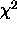subject to the constraint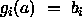. One way of achieving this is to add an extra term to the minimization cost function and the result is: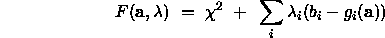where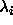are known as the Lagrange multipliers. These techniques must be used with care as the last thing we would wish to do is to trade off an arbitrary weighting of the inability to satisfy a constraint against the statistical measure we know we should be legitimately minimising. The optimization process is thus generally constructed such that eachhas reduced to zero by the the end. The Lagrange approach considerably complicates the estimation of additional information such as covariances (described below) and a discussion of these techniques is really beyond the scope of this tutorial. The reader is directed instead to  and we will only point out here that in many cases constraint equations can be satisfied directly by careful formulation of the model parameterisation a. For example, positive only parameters can be enforced by defining a parameter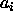instead as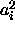in the model.

Another example of such a process is the definition of a rotation matrix which has nine parameters but only three degrees of freedom. This can be represented instead as a quaternion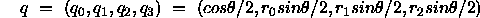where r defines an axis of rotation and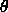the rotation about that axis. Only three of these parameters are defined for minimization and the other calculated in order to satisfy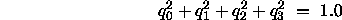Bob Fisher
Fri Mar 28 14:12:50 GMT 1997